Apr 3rd 2014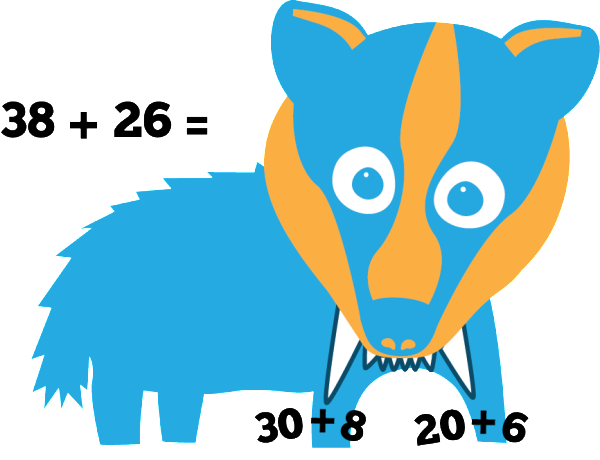Hi, I’m Brian Breaking Badger, and I love to break numbers apart.
Separating place value is considered my art!
I break numbers into ones and tens with my teeth,
Then I work with the place value underneath.
I’ll add or subtract the tens, then the ones.
Before you know it, the problem’s all done!

WHAT is breaking apart? Students use place value knowledge to decompose or break each number apart into hundreds, tens and ones.  Depending on the problem, students will either add or subtract each place value (first hundreds, then tens, and finally ones).  Students will then add or subtract all numbers to solve the problem. Read more about Brian’s strategy below or download the complete unit here.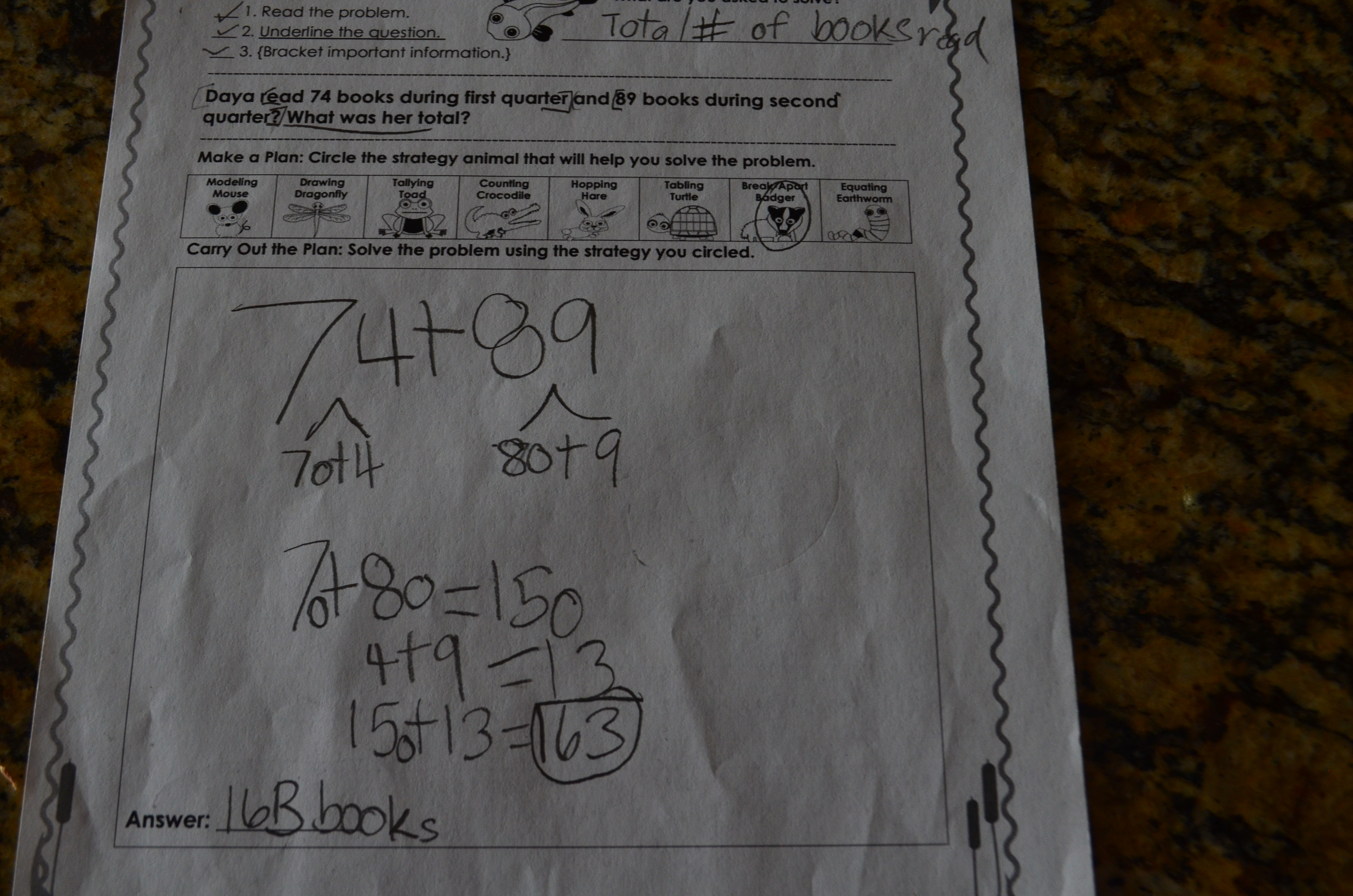WHY is breaking apart important?  When students use the breaking apart strategy, they are decomposing numbers by place value. This help helps strengthen mental computation, builds number sense and solidifies foundational place value skills. It also serves as an efficient method to double-check solutions as students.

HOW do I teach breaking apart? Teach breaking in isolation first so that students become familiar with the process of decomposing numbers. After proficiency is demonstrated, students can apply this strategy with story problems.

WHEN should I use breaking apart? This is an ideal strategy for developing mathematicians who have a solid place value and number sense foundation. It is most effective to introduce the breaking apart strategy after students are proficient with the hopping strategy since hopping requires place value identification and decomposition skills.

Provide place value and expanded notation practice. Students need a strong place value foundation to decompose or break apart numbers.  Provide regular practice through center games and kinesthetic activities. Simple games such as “Race to 100” where students roll dice and add numbers using place value manipulatives are engaging and effective.  This game is the perfect addition to weekly math centers.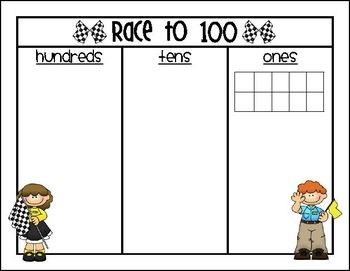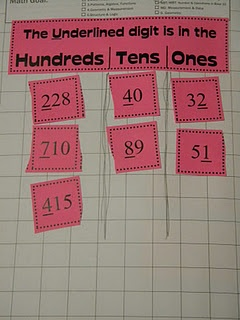Act out the problem.  Increase students’ understanding of the mathematical context by acting out the story problem.  Students also love to show their badger fangs when they break apart the numbers.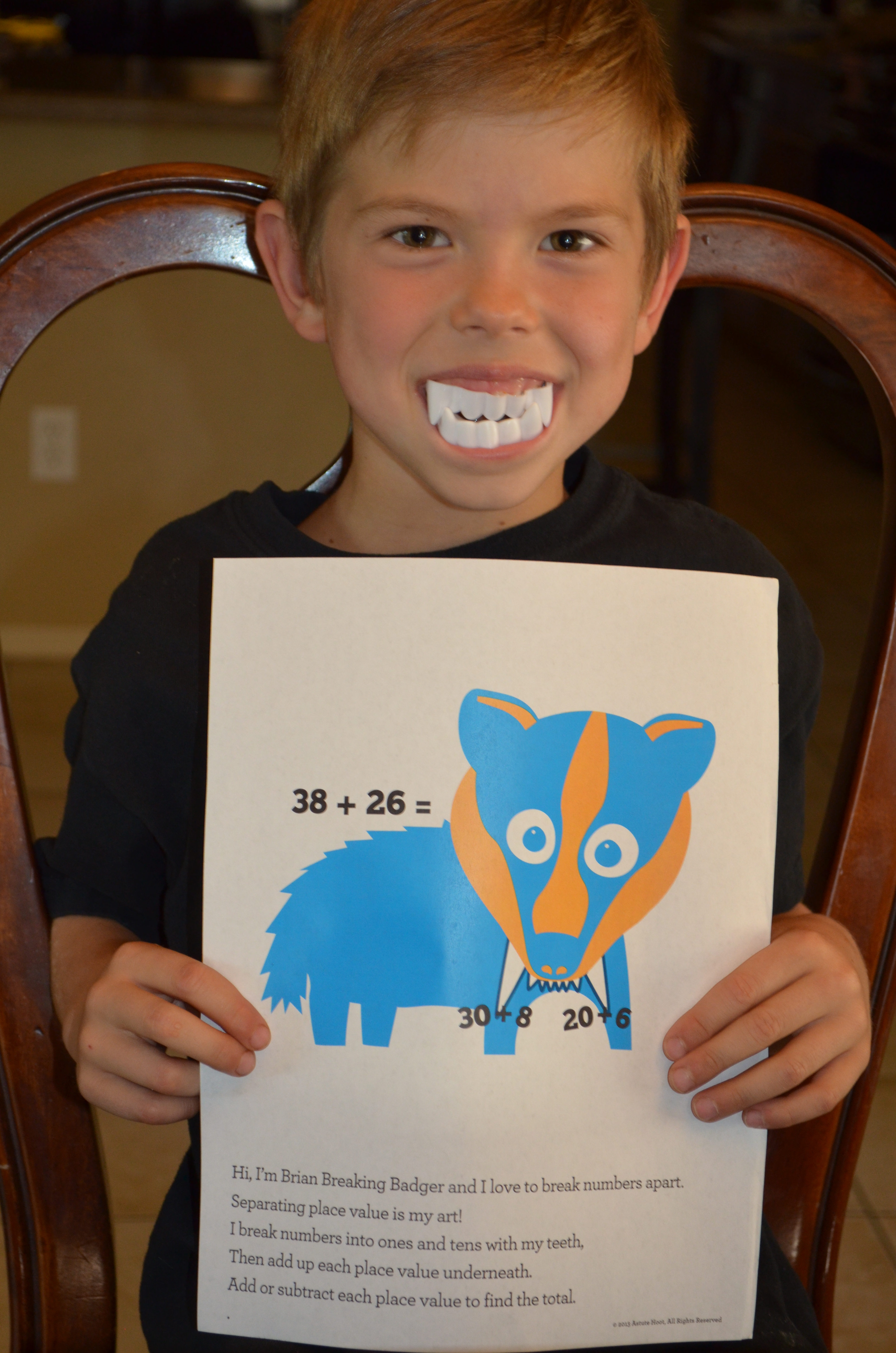Top